# Math in Focus Grade 1 Chapter 10 Practice 2 Answer Key Finding the Weight of Things

Practice the problems of Math in Focus Grade 1 Workbook Answer Key Chapter 10 Practice 2 Finding the Weight of Things to score better marks in the exam.

## Math in Focus Grade 1 Chapter 10 Practice 2 Answer Key Finding the Weight of Things

Fill in the blanks.

Example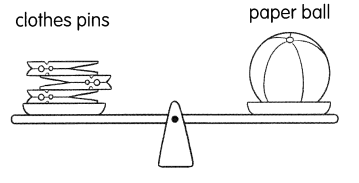The weight of the paper ball is about 3 clothes pins.

Question 1.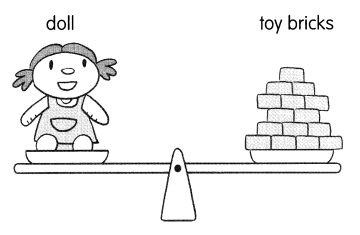The weight of the doll is about ____ toy bricks.

Question 2.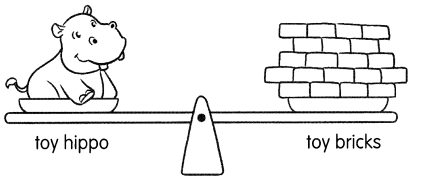The weight of the toy hippo is about ____ toy bricks.
Answer: The weight of the toy hippo is about 20 toy bricks.

Question 3.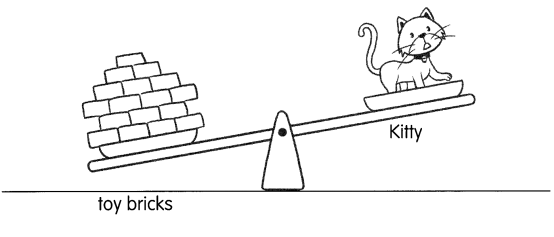The weight of Kitty is more than 15 toy bricks. The weight of Kitty is about ____ toy bricks.

Look at the pictures Then fill in the blanks.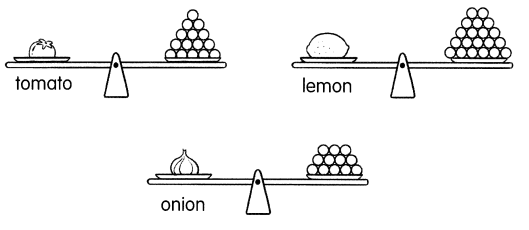Question 4.

Question 5.

Question 6.

Question 7.
The tomato is heavier than the ____
Answer: The tomato is heavier than the weight of onion.

Question 8.
The tomato is lighter than the ____
Answer: The tomato is lighter than the weight of lemon.

Question 9.
The ___ is the heaviest.
Answer: The weight of the lemon is the heaviest.

Question 10.
The ____ is the lightest.
Answer: The weight of the onion is the lightest.

Look at the pictures. Then fill in the blanks.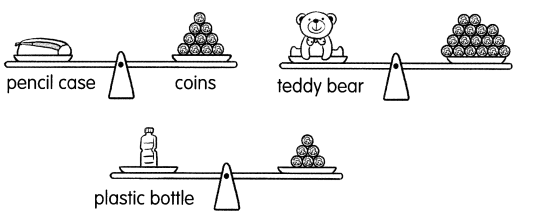Question 11.
The weight of the pencil case is ___ coins.
Answer: The weight of the pencil case is 10 coins.

Question 12.
The weight of the plastic bottle is ___ coins.
Answer: The weight of the plastic bottle is 6 coins.

Question 13.
The weight of the teddy bear is ___ coins.
Answer: The weight of the teddy bear is 17 coins.

Question 14.
The ____ is heavier than the plastic bottle.
Answer: The weight of the pencil case is heavier than the plastic bottle.

Question 15.
The ____ is lighter than the teddy bear.
Answer: The weight of pencil case is lighter than the teddy bear.

Question 16.
The pencil case is heavier than the ___
Answer: The pencil case is heavier than the plastic bottle.

Question 17.
The ____ is lighter than the ____
Answer: The pencil case is lighter than the teddy bear.

Question 18.
The heaviest thing is the ____
Answer: The heaviest thing is the teddy bear.

Question 19.
The lightest thing is the ____
Answer: The lightest thing is the plastic bottle.